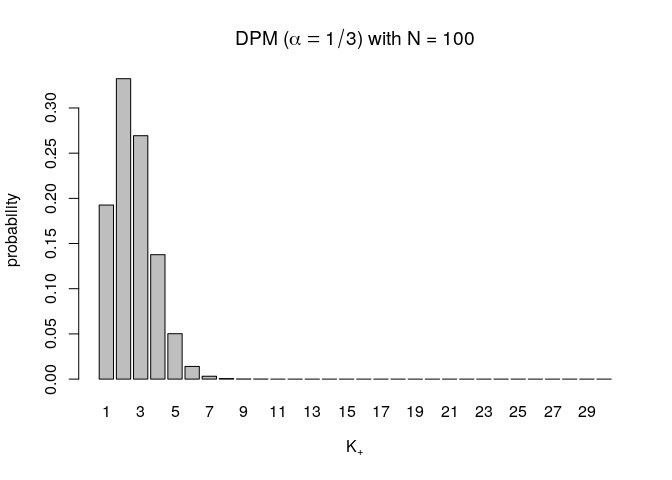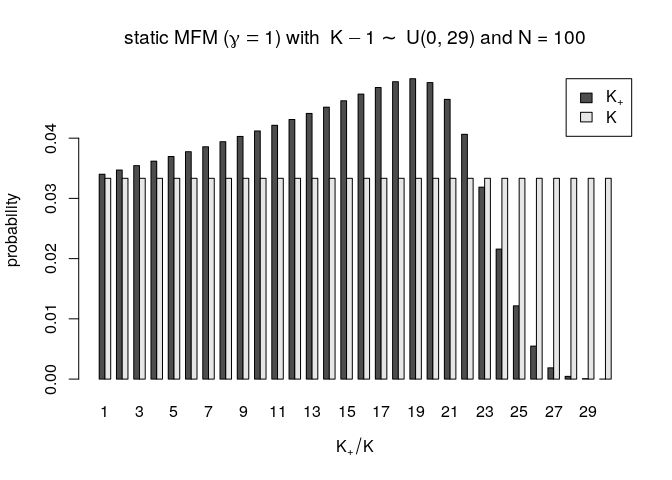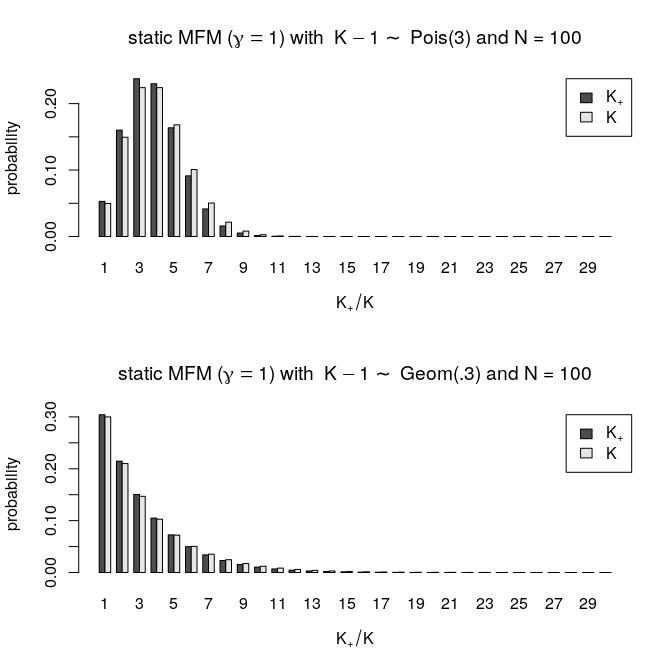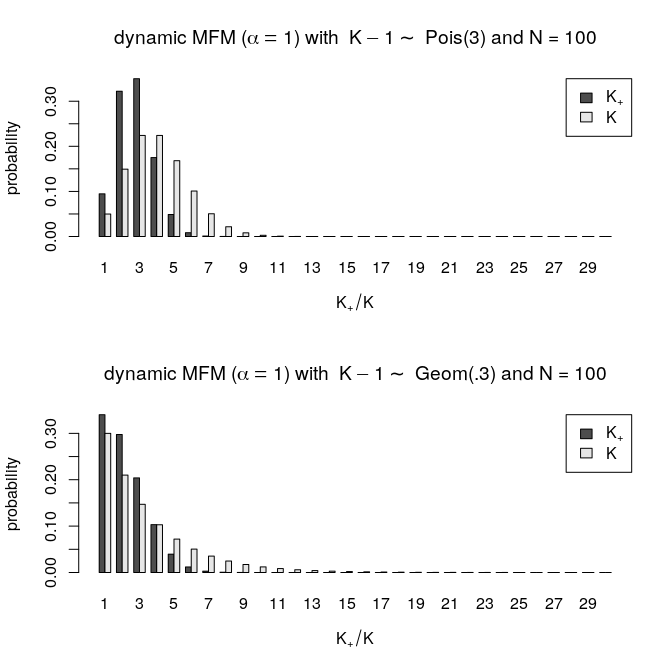Introduction

The name of the fipp package is an acronym for “functionals for implicit prior partitions” and the package mainly provides users with tools that can be used to inspect implicit assumptions on the prior distribution of partitions of a mixture model. This implies that the package is mostly tailored towards applied Bayesian statisticians working on clustering, mixture modeling and related areas (if you are using packages such as BayesMix and PReMiuM, you are the right audience). Specifically, it covers models that assign a prior distribution on the number of components in the mixture model such as Dirichlet Process Mixtures and models broadly classified as Mixture of Finite Mixtures (MFMs, as coined by Miller and Harrison (2018)).

Nonetheless, we believe some of the functionality may also be of interest to researchers in the broad area of mixture models, such as those who prefer to use an EM-based approach, most notably implemented in the mclust package.

This vignette is designed as a stand-alone and self-contained tour of the fipp package, although we suggest the interested reader to have a look at our paper Greve et al. (2020) for further understanding.

Functionality

Models considered in this package

So far, the package includes three important Bayesian mixture models that assign a prior on the number of components commonly denoted as $$K$$ as well as on the concentration parameter or the parameter for the Dirichlet distribution either denoted as $$\alpha$$ or $$\gamma$$. The Dirichlet parameter induces a sequence $$\gamma_K$$ for each $$K$$.

The three models considered are: Dirichlet Process Mixtures (DPMs), Static Mixtures of Finite Mixtures (Static MFMs) and Dynamic Mixtures of Finite Mixtures (Dynamic MFMs).

The important parameters for these models, $$K$$ and $$\gamma_K$$, are specified as follows:

DPM Static MFM Dynamic MFM
$$K$$ $$K = \infty$$ $$K-1\sim P(K)$$
$$K=1,2,\ldots$$
$$K-1\sim P(K)$$
$$K=1,2,\ldots$$
$$\gamma_K$$ $$\alpha$$ $$\gamma$$ $$\alpha/K$$

The concentration or Dirichlet parameter, regardless of the model, is usually given a prior, such as a gamma distribution which has support on the positive real line.

For the Static and Dynamic MFMs, $$P(K)$$ must be a distribution with support on the nonnegative integers. Following the suggestion in Frühwirth-Schnatter, Malsiner-Walli, and Grün (2020), the package implements the translated prior which specifies the prior on $$K-1$$ with support on the nonnegative integers.

An introduction to the theory behind the model and parameters

All three models considered in this package deal with mixture models which may be written as

\begin{align} p(y_i) = \sum^{K}_{k=1}\eta_kf_{\tau}(y_i|\theta_k) \end{align}

for observations $$y_i,i = 1,2,\ldots N$$.

Parameters $$\eta_k$$’s are weights that sum up to one, while $$f_{\tau}(\cdot|\theta)$$ refers to the component density which follows a distribution $$\tau(\theta)$$.

Furthermore, $$\eta_k$$’s are drawn from the symmetric Dirichlet distribution as follows:

\begin{align} \pmb{\eta}_K|K,\gamma_K \sim Dir_K(\gamma_K,\gamma_K,\ldots,\gamma_K) \end{align}

where $$\pmb{\eta}_K = (\eta_1,\eta_2,\ldots,\eta_K)$$.

An important quantity hidden in the equations above is the number of data clusters $$K_+$$ defined as

\begin{align} K_+ = K - \#\{\text{components k not associated to any y_i}\}. \end{align}

In other words, $$K_+$$ is the number of groups in the partition that separates $$y_i,\ i= 1,2,\ldots,N$$. Crucially, when we talk about a “k-cluster solution” for a data set, we refer not to $$K$$ but rather to $$K_+$$ being equal to $$k$$. Thus, we believe that $$K_+$$ and characteristics of the partitions captured through some functionals are more practically relevant quantities when specifying suitable priors for a Bayesian cluster analysis than $$K$$, and thus prior specifications regarding those should be done appropriately in some way.

To address this, the central aim of the fipp package is to translate the prior specifications with respect to $$K$$ and the concentration or Dirichlet parameter $$\gamma_K$$ into the number of clusters $$K_+$$ and various functionals computed over the partitions.

One can use results obtained from this package to conduct a sanity check on prior specifications w.r.t. $$P(K)$$ and $$\gamma_K$$ in terms of the implied distribution on $$K_+$$ and moments of functionals computed over the induced partitions.

Demo

Demonstration 1: induced prior on the number of clusters

First, we demonstrate how the fipp package can be used to obtain a prior pmf on the number of clusters $$K_+$$ from specifically selected $$P(K)$$ and $$\gamma_K$$. First, let’s load the package.

The function (to be precise, it is a closure as it returns a function) in question is called nClusters().

Let’s start with the implied number of clusters $$K_+$$ for a DPM with the concentration parameter $$\alpha = 1/3$$ (based on the recommendation in Escobar and West (1995)) and a sample size of $$N = 100$$. We evaluate the pmf between $$K_+ = 1$$ to $$K_+ = 30$$. Since $$K$$ is fixed to $$\infty$$ in DPMs, there is no prior on the number of components $$P(K)$$ to compare the resulting distribution on $$K_+$$ to.As we can see, this specification implicitly implies that the probability of $$K_+$$ being above 10 is very small. Also, the induced prior on $$K_+$$ generally prefers a sparse solution of $$K_+$$ with values between 1 and 3 for $$N = 100$$ observations and the probability of homogeneity (that is the probability of $$K_+ = 1$$) is about 1/5.

Now, let’s see what kind of inference we can draw for the Static MFM. Here, we replicate the specification used in Richardson and Green (1997) with $$U(1,30)$$ for $$K$$ (which translates to $$K-1 \sim U(0,29)$$) and $$\gamma = 1$$ for the concentration parameter.

This prior appears uninformative as we assume that $$K$$ is uniformly distributed on $$[1, 30]$$ and $$\gamma = 1$$ also corresponds to the uniform distribution on the simplex. However, it turns out to be still informative for $$K_+$$ as we can clearly see from the result below.While the distribution on $$K$$ is uniform and thus uninformative, that of $$K_+$$ induced by the uniform distribution on $$K$$ and $$\gamma = 1$$ is not uninformative. It has a clear peak around 19. This highlights the well known problem of using an uniform distribution for $$P(K)$$. When the sample size is relatively small, it gives a false sense of uninformativeness despite specifying a prior with a clear peak (for higher $$N$$ it does get more and more uniformly distributed).

Click here for a brief explanation of why this happens. There are several factors that are in play to produce the above result. Crucially, the higher the concentration parameter $$\gamma$$, the more likely it is that all $$K$$ components are filled, with many components being filled even for the relatively small sample size $$N$$. Therefore, despite the small $$N$$, $$K_+ \approx K$$ and thus the induced prior on $$K_+$$ also approximates the specified $$P(K)$$ (one can check this with the code above by increasing $$\gamma$$ to values such as 100). The reverse happens when $$\gamma$$ is small and the result obtained for the DPM more resembles results obtained for a Static MFM with a small $$\gamma$$ value.

The above example provides insights into the well known fact that the seemingly innocent choice of specifying a uniform prior on $$K$$ and the component weights $$\pmb{\eta}_K$$ results in a rather problematic behavior in terms of the prior on $$K_+$$ and could also skew the resulting posterior (see Nobile (2004) for details). In recent work on MFMs different alternative distributions with support on $$\mathbb{Z}_{>0}$$ have been suggested.

Again, we can use the function returned by the closure nClusters() to inspect several choices of such $$P(K)$$’s. The resulting function allows computations shared across all $$P(K)$$’s to only be run once regardless of the number of $$P(K)$$’s considered. Here we examine two choices: $$Pois(3)$$ and $$Geom(0.3)$$.Similarly, we can do the same comparison w.r.t. the Dynamic MFM with $$\alpha = 1$$ (note that this is not related in any way to the Static MFM with $$\gamma = 1$$). Again, computations shared between these $$P(K)$$’s will not be re-run, thus allowing users to experiment with multitudes of $$P(K)$$’s in practical applications.Here, the distributions on $$K_+$$ and $$K$$ do not coincide, especially for the Poisson case. We leave the details to the paper Frühwirth-Schnatter, Malsiner-Walli, and Grün (2020). To put it simply, as the concentration parameter is now $$\alpha/K$$, greater values of $$K$$ induce smaller values $$\alpha/K$$ and $$K_+$$ tends to be less than $$K$$, thus skewing the distribution of $$K_+$$ more towards the right than that of $$K$$.

For further details concerning the difference between the Static and Dynamic MFM w.r.t. the induced prior on $$K_+$$, we refer to Frühwirth-Schnatter, Malsiner-Walli, and Grün (2020) and Greve et al. (2020).

Demonstration 2: relative entropy of the induced prior partitions

Another important function (again it is a closure to be precise) available in the package is fipp(). It allows the user to compute moments (currently only up to the 2nd moment) of any additive symmetric functional over the induced prior partitions specified through picking $$P(K)$$, $$\gamma_K$$ and $$K_+$$.

Here, we pick the relative entropy as functional which is given by

$-\frac{1}{\log(K_+)}\sum_{i=1}^{K_+}\frac{N_i}{N}\log\Bigg(\frac{N_i}{N}\Bigg)$

with $$K_+$$ the number of clusters and $$N_i$$ the number of observations in the $$i$$-th cluster. This functional takes values between $$(0,1)$$ and the closer this functional is to 1, the more evenly distributed the $$N_i$$’s are and vise versa.

Let’s start with the DPM with $$\alpha = 1/3$$ as before and with the specific case where $$K_+ = 4$$. Function fipp() determines the functional based on the sum of the results of a vectorized function of the induced unordered cluster sizes $$(N_i)_{i=1,\ldots,K_+}$$. This implies that the functional must be additive and symmetric. In addition, the vectorized function must be supplied in its log form for computational reasons.

Therefore, for the relative entropy, the vectorized function supplied to fipp() determines $$\log(N_i)-\log(N)+\log(\log(N)-\log(N_i))$$. The resulting prior mean and standard deviation of the functional (default specification returns mean/variance, which can be changed to 1st/2nd moments) will be divided by $$\log(K_+)$$ and $$\log(K_+)^2$$, respectively.

## Statistics computed over the prior partitions: Relative entropy
##  Model: DPM (alpha = 1/3)
##  conditional on: K+ = 4
##  mean = 0.5654722
##  sd = 0.1987301

It turns out that the value of the concentration parameter $$\gamma_K \equiv \alpha$$ does not play any role in the relative entropy of the prior partitions for DPMs (try adjusting alpha = and see for your self) nor does $$P(K)$$ as $$K$$ is fixed to $$\infty$$. Thus, DPM induces a fixed structure a-priori on the partitions conditional on $$K_+$$.

Now let’s see what the prior mean and standard deviation of the relative entropy of the Static MFM with $$\gamma = 1$$ and two priors on $$K-1$$ ($$Pois(3)$$ and $$Geom(0.3)$$) conditional on $$K_+ = 4$$ is.

## Statistics computed over the prior partitions: Relative entropy
##  Model: static MFM (gamma = 1)
##  conditional on: K+ = 4
##  case 1 with K-1 ~ dpois(3): mean = 0.7920192  sd = 0.1310269
##  case 2 with K-1 ~ dgeom(.3): mean = 0.7920192  sd = 0.1310269

Here, we observe that the choice of $$P(K)$$ does not affect the relative entropy of the induced prior partitions for the Static MFM. Nonetheless, the choice of $$\gamma_K\equiv \gamma$$ will still affect the relative entropy unlike the DPM.

Now, let’s see what the Dynamic MFM with $$\alpha = 1$$ and two priors on $$K-1$$ ($$Pois(3)$$ and $$Geom(0.3)$$) conditional on $$K_+ = 4$$ gives.

## Statistics computed over the prior partitions: Relative entropy
##  Model: dynamic MFM (alpha = 1)
##  conditional on: K+ = 4
##  case 1 with K-1 ~ dpois(3): mean = 0.6337794  sd = 0.1860941
##  case 2 with K-1 ~ dgeom(.3): mean = 0.6269217  sd = 0.1879739

As we can see, the relative entropy of the induced prior partitions for the Dynamic MFM depends on both the concentration parameter $$\gamma_K\equiv \alpha$$ as well as the prior on $$K$$. Therefore, it is the most flexible of the three models considered when it comes to embedding prior information regarding the characteristics of the partitions captured through relative entropy.

Demonstration 3: expected number of clusters which contain less than $$10\%$$ of the observation each

Now let’s consider other functionals. For example, we may be interested in the a-priori expected number (and corresponding standard deviations) of clusters which contain less than $$10\%$$ of the observations.

The vectorized function for this functional required by fipp() can simply be written as $$\log(\mathbb{I}_{N_i<0.1*N})$$ where $$\mathbb{I}_{.}$$ stands for the indicator function. Let’s reuse the specifications in Demo 2 by only replacing the functional. We do not show the code for the sake of conciseness.

## Statistics computed over the prior partitions:
##  Number of clusters with less than 10% of the obs
##  Model: DPM (alpha = 1/3)
##  conditional on: K+ = 4
##  mean = 1.880596
##  sd = 0.780884
## Statistics computed over the prior partitions:
##  Number of clusters with less than 10% of the obs
##  Model: static MFM (gamma = 1)
##  conditional on: K+ = 4
##  case 1 with K-1 ~ dpois(3): mean = 1.003997  sd = 0.7399482
##  case 2 with K-1 ~ dgeom(.3): mean = 1.003997  sd = 0.7399482
## Statistics computed over the prior partitions:
##  Number of clusters with less than 10% of the obs
##  Model: dynamic MFM (alpha = 1)
##  conditional on: K+ = 4
##  case 1 with K-1 ~ dpois(3): mean = 1.646882  sd = 0.793471
##  case 2 with K-1 ~ dgeom(.3): mean = 1.670937  sd = 0.7938899

We see that specifications used for DPMs and Dynamic MFMs result in expectation in about 2 clusters out of 4 having less than $$10\%$$ of the observations. This indicates rather unevenly sized partitions which is in line with the relative entropy results derived earlier.

The Static MFM with $$\gamma=1$$ seems to produce partitions that are more evenly distributed with the expected number of clusters containing less than $$10\%$$ observations being close to 1. Again, this aligns with the relative entropy results obtained for the Static MFM earlier.

Other symmetric additive functionals that might be of interest, such as the expected number of singleton clusters ($$\mathbb{I}_{N_i = 1}$$), might also easily be used together with the fipp() function.